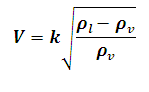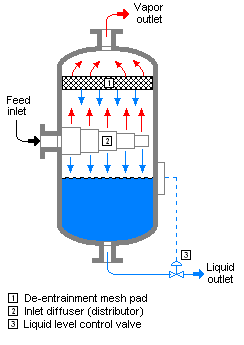# Souder's-Brown Calculator

This Calctown Calculator calculates the maximum allowable vapor velocity according to the souder's-brown equation.

m/s
kg/m3
kg/m3

#### Result

m/sClick here to view image

where

V = maximum allowable vapor velocity

k = vapor velocity factor

ρl = liquid density

ρv = vapor density